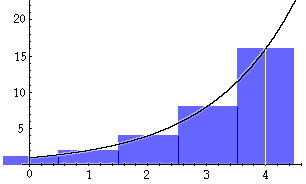Subject: Integral Calculus problem

Dear Sir:

A fellow teacher asked me about a problem she wanted to give to her students. It involved whether to take a million dollars or a penny doubled a number of times. I was able to determine the number must have been .01 * 230 which is about \$10 million and a lot more than \$1 million. Then I reasoned, what about the doubling from 1 - 29. I reasoned that since each amount would be half the previous, the total amount would be the same as

.01 * 231 - .01 = \$21,474,836.47.

To check that I was right I used a spreadsheet and did a Riemann sum.

 1 \$0.01 2 \$0.02 3 \$0.04 4 \$0.08 5 \$0.16 . . . . . . 29 \$5,368,709.12 30 \$10,737,418.24 _________________ Total \$21,474,836.47

When I finished I reasoned that I had done the task in several steps and I could have done it in 1 step. Thus I integrated .01 * 2x from 0 through 30 but the number I got was \$15,490,820.0324. [.01 * (1/ln 2)(230 - 20)]. I then used the finite integral on my graphing calculator and got the same number. I have been unable to figure out why I don't get the correct answer by integration. I have asked a couple of other math teachers but no one has been able to give me an answer.

Yours truly,

Douglas Norberg

Hi Douglas,

I want to do this with fewer time intervals (only 5) and make the units cents rather than dollars to eliminate the decimals. This gives

 1 01 2 02 3 04 4 08 5 16 _________________ Total 31

1 * 25 - 1 = 31

In this case the integral of 1 * 2x from 0 through 4 is 21.64.

The cause of the discrepancy can be seen in the diagram. The blue rectangles each have a base of 1 unit and a heights of 20 = 1, 21 = 2, 22 = 4, 23 = 8, and 24 = 16, for a total area of 31 square units. However the integral of 1 * 2x from 0 through 4 is is the area under the curve in black from 0 to 4; a value of 21.64.The largest difference between the two areas is the strip of the last rectangle from 4 to 4.5. If you integrate 1 * 2x from 0 through 4.5 you get 31.20, much closer to 31.

In your original problem the strip under the curve from 30 to 30.5 is very large. When I calculated the integral of .01 * 2x from 0 through 30.5 I got \$21,907,327.79.

I hope this helps,
Harley
Go to Math Central